## Basic Log Amplifier Using Diode:

The circuit diagram of basic log amplifier using a diode is shown in the Fig. 2.69.The diode D is used in the negative feedback path. The node A is grounded hence node B is at virtual ground. Hence VB = 0.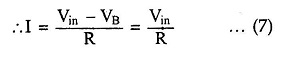As the op-amp input current is zero,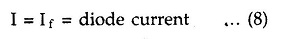Now If is the current through diode and voltage across diode is VB – Vo i.e.-Vo.

Hence using equation (10) we can write,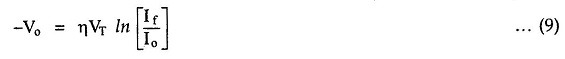Substituting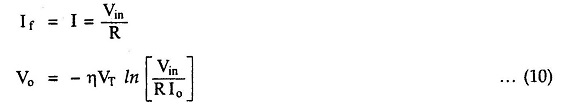As Io R is constant d.c.voltage, let us denote it as Vref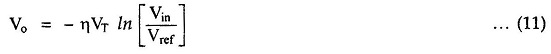Where

•  Vref = Io R

Thus the output voltage Vo is a function of logarithm of the input voltage Vin. The Basic Log Amplifier Using Diode gives the logarithm to base e i.e., natural logarithm. But the same circuit can be used to find out logarithm values to base 10 by the proper scaling as,